Next: Dendrites with the closed Up: Dendrites Previous: Dendrites of de Groot-Wille

## Modified Miller dendrites

The methods used by Miller to construct his examplehas also been applied to get a modification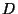ofin [Charatonik 1979, Chapter IV, p. 230] (an outline of this construction is in [Caratonik 1977]). Below we summarize properties of this example, and recall an extension of this construction.

1. There exists a dendritesuch that:
1. each point ofis of order nor greater than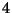;
2. for each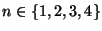the set of all points ofwhich are of order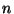is dense in;
3.is strongly rigid;
4.is chaotic.
2. For any two integers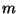andwith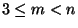there exists a dendrite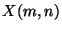such that (see [Charatonik et al. 1996, Theorem 5.5, p. 185] and compare also [Charatonik 1999, Theorem 27, p. 24]):
1.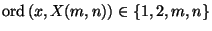for each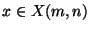.
2. Every arc incontains a point of orderin.
3. Each of the sets: of all end points of, of all points of orderinand of all points of orderinis dense in.
4.is strongly chaotic.

Dendriteis called a modified Miller dendrite . (For a further modification of the above construction that leads to chaotic, strongly rigid, openly rigid and not strongly chaotic dendrites, see [Charatonik 2000, Theorem 3.14, p. 650].)

Finally recall dendrites constructed in [Charatonik 1999, Examples 33 and 35, p. 28].

3. For each natural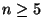, there exists a strongly rigid and not chaotic dendrite, all points of which are of order at most([Charatonik 1999, Example 33, p. 28]).
4. There exists a rigid dendrite which is neither chaotic nor strongly rigid ([Charatonik 1999, Example 35, p. 28]).

Here you can find source files of this example.

Here you can check the table of properties of individual continua.

Here you can read Notes or write to Notes ies of individual continua.Next: Dendrites with the closed Up: Dendrites Previous: Dendrites of de Groot-Wille
Janusz J. Charatonik, Pawel Krupski and Pavel Pyrih
2001-11-30